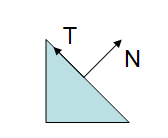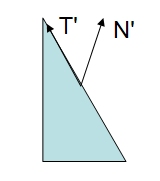Prev: Multi-Texture Next: Normalization Issues

The gl_NormalMatrix is present in many vertex shaders. In here some light is shed on what is this matrix and what is it for. This section was inspired by the excellent book by Eric Lengyel “Mathematics for 3D Game Programming and Computer Graphics”.

Many computations are done in eye space. This has to do with the fact that lighting is commonly performed in this space, otherwise eye position dependent effects, such as specular lights would be harder to implement.

Hence we need a way to transform the normal into eye space. To transform a vertex to eye space we can write:

`	vertexEyeSpace = gl_ModelViewMatrix * gl_Vertex;`

So why can’t we just do the same with a normal vector? A normal is a vector of 3 floats and the modelview matrix is 4×4. Secondly, since the normal is a vector, we only want to transform its orientation. The region of the modelview matrix that contains the orientation is the top left 3×3 submatrix. So why not multiply the normal by this submatrix?

This could be easily achieved with the following code:

`	normalEyeSpace = vec3(gl_ModelViewMatrix * vec4(gl_Normal,0.0));`

So, gl_NormalMatrix is just a shortcut to simplify code writing or to optimize it? No, not really. The above line of code will work in some circumstances but not all.

Lets have a look at a potential problem:;

In the above figure we see a triangle, with a normal and a tangent vectors. The following figure shows what happens when the modelview matrix contains a non-uniform scale.Note: if the scale was uniform, then the direction of the normal would have been preserved, The length would have been affected but this can be easily fixed with a normalization.

In the above figure the Modelview matrix was applied to all the vertices as well as to the normal and the result is clearly wrong: the transformed normal is no longer perpendicular to the surface.

We know that a vector can be expressed as the difference between two points. Considering the tangent vector, it can be computed as the difference between the two vertices of the triangle’s edge. If$P_1$ and$P_2$ are the vertices that define the edge we know that:

`$T = P_2 - P_1$$T = P_2 - P_1$`

Considering that a vector can be written as a four component tuple with the last component set to zero, we can multiply both sides of the equality with the Modelview matrix

`$T * Modelview = (P_2-P_1)* Modelview$$T * Modelview = (P_2-P_1)* Modelview$`

This results in

```$T * Modelview = P_2 * Modelview - P_1 * Modelview$$T * Modelview = P_2 * Modelview - P_1 * Modelview$$T' = P_2' - P_1'$$T' = P_2' - P_1'$```

As$P_1'$ and$P_2'$ are the vertices of the transformed triangle,$T'$ remains tangent to the edge of the triangle. Hence, the Modelview preserves tangents, yet it does not preserve normals.

Considering the same approach used for vector T, we can find two points$Q_1$ and$Q_2$ such that

`$N = Q_2 - Q_1$$N = Q_2 - Q_1$`

The main issue is that the a vector defined through the transformed points,$Q_2' - Q_1'$, does not necessarily remain normal, as shown in the figures above. The normal vector is not defined as a difference between two points, as the tangent vector, it is defined as a vector which is perpendicular to a surface.

So now we know that we can’t apply the Modelview in all cases to transform the normal vector. The question is then, what matrix should we apply?

Consider a 3×3 matrix G, and lets see how this matrix could be computed to properly transform the normal vectors.

We know that, prior to the matrix transformation T.N = 0, since the vectors are by definition perpendicular. We also know that after the transformation N’.T’ must remain equal to zero, since they must remain perpendicular to each other. T can be multiplied safely by the upper left 3×3 submatrix of the modelview (T is a vector, hence the w component is zero), let’s call this submatrix M.

Let’s assume that the matrix G is the correct matrix to transform the normal vector. T. Hence the following equation:

`$N' . T' = (GN) . (MT) = 0$$N' . T' = (GN) . (MT) = 0$`

The dot product can be transformed into a product of vectors, therefore:

`$(GN).(MT) = (GN)^T * (MT)$$(GN).(MT) = (GN)^T * (MT)$`

Note that the transpose of the first vector must be considered since this is required to multiply the vectors. We also know that the transpose of a multiplication is the multiplication of the transposes, hence:

`$(GN)^T (MT) = N^TG^TMT$$(GN)^T (MT) = N^TG^TMT$`

We started by stating that the dot product between N and T was zero, so if

`$G^TM = I$$G^TM = I$`

then we have

`$N'.T' = N.T = 0$$N'.T' = N.T = 0$`

Which is exactly what we want. So we can compute G based on M.

`$G^TM = I \Longleftrightarrow G = (M^{-1})^T$$G^TM = I \Longleftrightarrow G = (M^{-1})^T$`

Therefore the correct matrix to transform the normal is the transpose of the inverse of the M matrix. OpenGL computes this for us in the gl_NormalMatrix.

In the beginning of this section it was stated that using the Modelview matrix would work in some cases. Whenever the 3×3 upper left submatrix of the Modelview is orthogonal we have:

`$M^{-1} = M^T \Longrightarrow G = M$$M^{-1} = M^T \Longrightarrow G = M$ `

This is because with an orthogonal matrix, the transpose is the same as the inverse. So what is an orthogonal matrix? An orthogonal matrix is a matrix where all columns/rows are unit length, and are mutually perpendicular. This implies that when two vectors are multiplied by such a matrix, the angle between them after transformation by an orthogonal matrix is the same as prior to that transformation. Simply put the transformation preserves the angle relation between vectors, hence transformed normals remain perpendicular to tangents! Furthermore it preserves the length of the vectors as well.

So when can we be sure that M is orthogonal? When we limit our geometric operations to rotations and translations, i.e. when in the OpenGL application we only use glRotate and glTranslate and not glScale. These operations guarantee that M is orthogonal. Note: gluLookAt also creates an orthogonal matrix!

 Prev: Multi-Texture Next: Normalization Issues

### 20 Responses to “The Normal Matrix”

1.Hi, you just make the calculated normal vector perpendicular to the tangent vector, but the result may have two direction. Similarly, -result(your result) is also make it perpendicular. Doesn’t really matter?

•Hi,

In this case it doesn´t matter since the dot product only considers the smallest angle between the two vectors, and we’re only interested in the situation where the vectors are perpendicular.

2.Very last mistake

“glRotate and gl_Translate and not glScale”

Why “gl_Translate” with with a under stroke?

And the rest of your articel is very well done.

•Many thanks!

3.Again a mistake:

“difference” instead of “diference”

“normal vector is not defined as a diference between two points”

4.Mistake?
“Considering the tangent vector, it can be computed as the different between”

–> difference instead of different

•Thanks.

5.Greate explanation, very thnks to author.

6.this tutorial explan clearly about why we can not directly adopt the modelViewMatrix for transforming a normal from object space to eye space , I benefit a lot from it ,thanks a lot !!

7.What if gl_scale is used to scale uniformly? SO that x,y, and z are all scaled together? Is the inverse and the tangent still the same? I’m actually not doing gl_scale but applying a uniform scaling transformation matrix.

void matrixUniformScale(float scale, mat4 m)
{
m = m = m = m = 0.0;
m = m = m = m = 0.0;
m = m = m = m = 0.0;
m = 1.0;
// Scale slots.
m = m = m = scale;
}

•Ahhh… you already answered it…

Note: if the scale was uniform, then the direction of the normal would have been preserved, The length would have been affected but this can be easily fixed with a normalization.

8.can u explain how to calculate “gl_normal” manually ? (For opengl Es gl_Normal is deprecated).

•Hi Vivek,

The gl_normal refers to the normal of each vertex. When you load a model these are usually part of it. To compute them yourself you must find a vector which is perpendicular to the surface you’re creating. The cross product is an operator that provides a perpendicular vector to the two input vectors, so its the way to go unless the model is defined by a function, in which case the there is usually an analytical process of computing the normal too. Using the dot product, just make two vectors from your triangle vertices and the result is a normal to the triangle. If the vertex is shared among triangles, just average all the normals of those triangles. Before you’re done you need to normalize it.

Hope this helps,

Antonio

9.I don’t get it, you said it is ok for T to be multiplied by M because it is a vector, although you said that it is not ok for N to be multilied by M, N is also a vector ? there is a contradiction in the explanation, which one is it ? in case we don’t have a uniform scale, why is it ok to multiply T by M and not OK for N, please explain this point … thank you

•Hi,

Thanks for the feedback. You’re right, the text could be confusing. I’ve updated the page hoping to make it more clear 🙂

10.nice explanation. thanks!

11.Very good. It is much clearer now. Thanks a million

12.To guarantee M is orthogonal, shouldn’t only rotations and scaling are to be allowed instead of rotations and translations?

•What I meant was diagonal in previous comment.
If translations are allowed why the 4×4 matrix will be orthogonal?

•The 4×4 matrix is not orthogonal as you point out, but the 3×3 top left submatrix is if no scales are applied. By setting the fourth component of the vector to zero and then considering only the vec3 of the result is the same as multiplying the the top left 3×3 sub matrix.

You’re right in your comments as the tutorial is not clear that I’m considering only the top left 3×3 submatrix when discussing orthogonality. The text has been updated, hopefully its clearer now.

Thanks for the feedback.

This site uses Akismet to reduce spam. Learn how your comment data is processed.